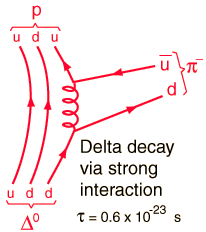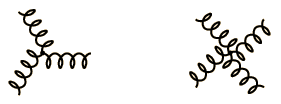# Feynman Diagrams and the Strong Force

At the most fundamental level, the strong force is an exchange force between quarks mediated by gluons. The use of Feynman diagrams to visualize the strong interaction involves primitive vertices with quarks and gluons. Quarks interact with each other by the exchange of gluons; a primitive vertex in the Feynman diagram involves a change in "color" and can take the formThe gluons carry the "color charge" and therefore the emergent quark will not have the same color as the entering quark. This process is very different from the electromagnetic force since the photon as the exchange particle for the force between charges does not itself carry charge. An interaction between quarks could be represented by the diagramThe interaction depicted here is responsible for binding quarks together into mesons and baryons, and responsible for holding protons and neutrons together to form nuclei.

 Particles can decay via the strong interaction, and if such a decay pathway is available to a particle, it decays very quickly - on the order of 10-23 seconds. An example is the decay Δ0 → p+ + π-. Note that the delta baryon Δ0 has the same quark makeup as the neutron, but its mass is much larger. Its mass is sufficient for this decay to be energetically favorable.Because gluons carry color, the property associated with the strong interaction, then they can interact with each other. Possible Feynman diagrams for those interactions areThis possibility of "glueballs" greatly increases the complexity of the strong interaction. In addition to bound quarks, there can be collections of bound gluons hanging around.

Index

Fundamental force concepts

Reference
Griffiths
Ch 2

 HyperPhysics***** Quantum Physics R Nave
Go Back